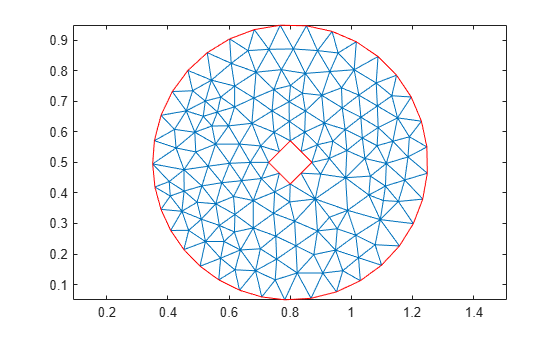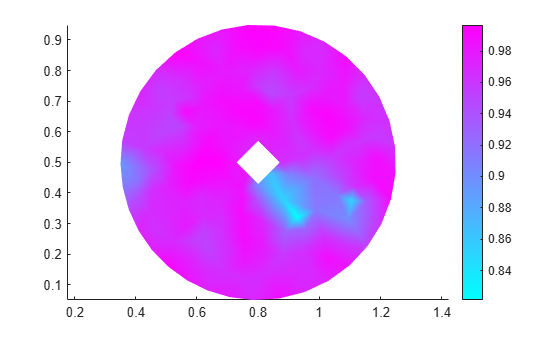pdetriq

(Not recommended) Triangle quality measure

pdetriq is not recommended. Use meshQuality instead.

Description

example

q = pdetriq(p,t) returns a row vector of numbers from 0 through 1 representing the triangle quality of all the elements of the [p,e,t] mesh.

pdetriq evaluates the quality of a triangle as

$q=\frac{4a\sqrt{3}}{{h}_{1}^{2}+{h}_{2}^{2}+{h}_{3}^{2}}$

where a is the area and h1, h2, and h3 are the lengths of the edges of the triangle.

The value 0 corresponds to a degenerate triangle with zero area. The value 1 corresponds to a triangle with h1 = h2 = h3.

Examples

collapse all

Evaluate the quality for each triangle of a [p,e,t] mesh.

Generate and plot a mesh for the geometry consisting of a circle with a diamond hole.

[p,e,t] = initmesh(@scatterg);
pdemesh(p,e,t)
axis equalEvaluate the triangle quality for each mesh triangle. Plot the resulting quality values.

q = pdetriq(p,t);
figure
pdeplot(p,e,t,'XYData',q);
axis equalInput Arguments

collapse all

Mesh nodes, specified as a 2-by-Np matrix of nodes (points), where Np is the number of nodes in the mesh. For details on the mesh data representation, see initmesh.

Data Types: double

Mesh elements, specified as a 4-by-Nt matrix of triangles, where Nt is the number of triangles in the mesh. For details on the mesh data representation, see initmesh.

Data Types: double

Output Arguments

collapse all

Triangle quality, returned as a row vector of numbers from 0 through 1.

 Bank, Randolph E. PLTMG: A Software Package for Solving Elliptic Partial Differential Equations, User's Guide 6.0. Philadelphia, PA: Society for Industrial and Applied Mathematics, 1990.

Partial Differential Equation Toolbox DocumentationGet trial now